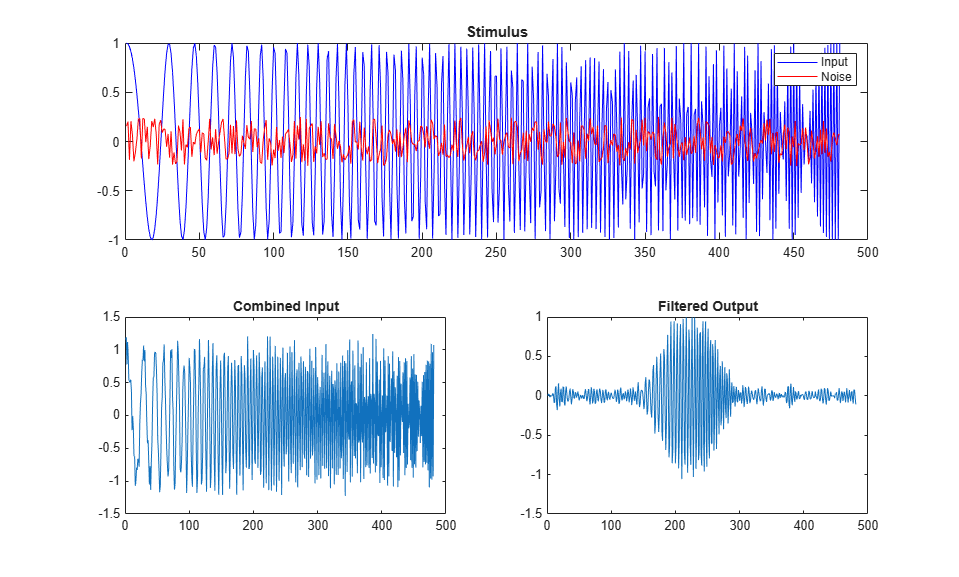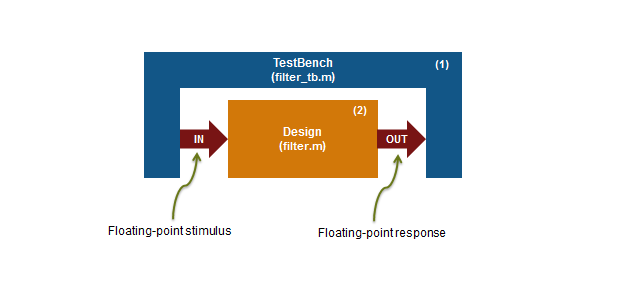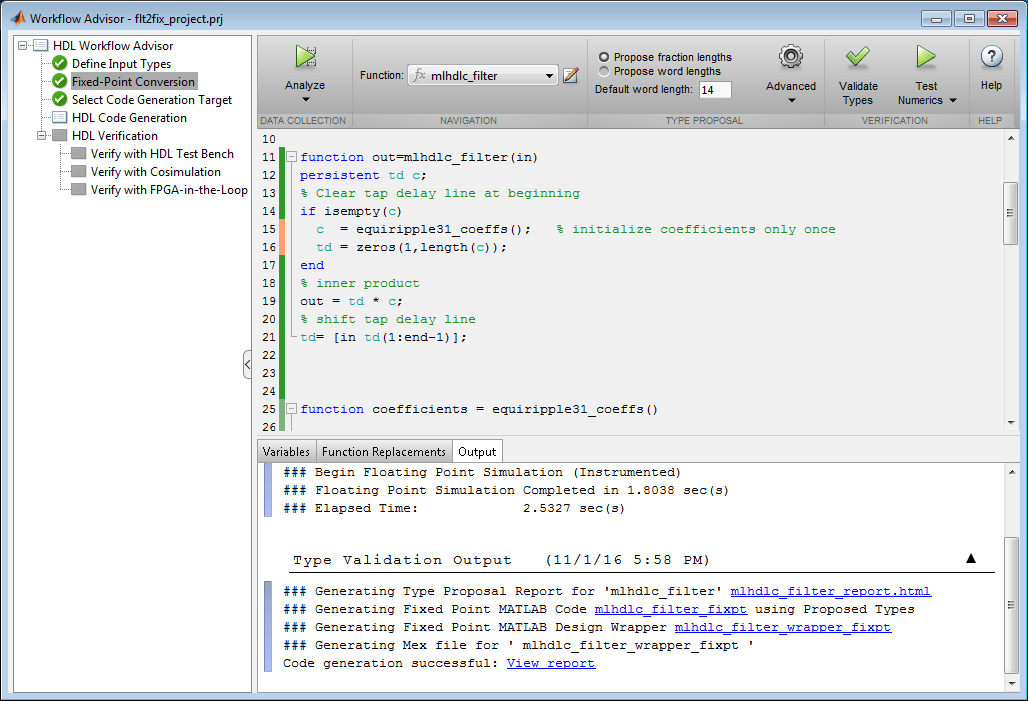# Working with Generated Fixed-Point Files

This example shows how to work with the files generated during floating-point to fixed-point conversion.

### Introduction

This tutorial uses a simple filter implemented in floating-point and an associated testbench to illustrate the file structure of the generated fixed-point code.

```design_name = 'mlhdlc_filter'; testbench_name = 'mlhdlc_filter_tb'; ```

### MATLAB® Code

1. MATLAB Design: mlhdlc_filter

2. MATLAB testbench: mlhdlc_filter_tb

### Create a New Folder and Copy Relevant Files

Executing the following lines of code copies the necessary example files into a temporary folder.

```mlhdlc_demo_dir = fullfile(matlabroot, 'toolbox', 'hdlcoder', 'hdlcoderdemos', 'matlabhdlcoderdemos'); mlhdlc_temp_dir = [tempdir 'mlhdlc_flt2fix']; % create a temporary folder and copy the MATLAB files cd(tempdir); [~, ~, ~] = rmdir(mlhdlc_temp_dir, 's'); mkdir(mlhdlc_temp_dir); cd(mlhdlc_temp_dir); copyfile(fullfile(mlhdlc_demo_dir, [design_name,'.m*']), mlhdlc_temp_dir); copyfile(fullfile(mlhdlc_demo_dir, [testbench_name,'.m*']), mlhdlc_temp_dir); ```

### Simulate the Design

Simulate the design with the testbench prior to code generation to make sure there are no runtime errors.

```mlhdlc_filter_tb ```### Create a New HDL Coder™ Project

To create a new project, enter the following command:

```coder -hdlcoder -new flt2fix_project ```

Next, add the file 'mlhdlc_filter' to the project as the MATLAB Function and 'mlhdlc_filter_tb' as the MATLAB Test Bench.

Refer to Get Started with MATLAB to HDL Workflow for a more complete tutorial on creating and populating MATLAB HDL Coder projects.

### Fixed-Point Code Generation Workflow

Perform the following tasks in preparation for the fixed-point code generation step:

2. Choose `Convert to fixed-point at build time` for the option Fixed-point conversion.

3. Right-click the Fixed-Point Conversion step and select Run to Selected Task to execute the instrumented floating-point simulation.

Refer to Floating-Point to Fixed-Point Conversion for a more complete tutorial on these steps.

### Floating-Point Design Structure

The original floating-point design and testbench have the following relationship.For floating-point to fixed-point conversion, the following requirements apply to the original design and the testbench:

• The testbench 'mlhdlc_filter_tb.m' (1) must be a script or a function with no inputs.

• The design 'mlhdlc_filter.m' (2) must be a function.

• There must be at least one call to the design from the testbench. All call sites contribute when determining the proposed fixed-point types.

• Both the design and testbench can call other sub-functions within the file or other functions on the MATLAB path. Functions that exist within matlab/toolbox are not converted to fixed-point.

In the current example, the MATLAB testbench 'mlhdlc_filter_tb' has a single call to the design function 'mlhdlc_filter'. The testbench calls the design with floating-point inputs and accumulates the floating-point results for plotting.

### Validate Types

During the type validation step, fixed-point code is generated for this design and compiled to verify that there are no errors when applying the types. The output files will have the following structure.The following steps are performed during fixed-point type validation process:

1. The design file 'mlhdlc_filter.m' is converted to fixed-point to generate fixed-point MATLAB code, 'mlhdlc_filter_fixpt.m' (3).

2. All user-written functions called in the floating-point design are converted to fixed point and included in the generated design file.

3. A new design wrapper file is created, called 'mlhdlc_filter_wrapper_fixpt.m' (2). This file converts the floating-point data values supplied by the testbench to the fixed-point types determined for the design inputs during the conversion step. These fixed point values are fed into the converted fixed-point design, 'mlhdlc_filter_fixpt.m'.

4. 'mlhdlc_filter_fixpt.m' will be used for HDL code generation.

5. All the generated fixed-point files are stored in the output directory 'codegen/mlhdlc_filter/fixpt'.Click the links to the generated code in the Workflow Advisor log Window to examine the generated fixed-point design and wrapper.

### Clean up the Generated Files

Run the following commands to clean up the temporary project folder.

```mlhdlc_demo_dir = fullfile(matlabroot, 'toolbox', 'hdlcoder', 'hdlcoderdemos', 'matlabhdlcoderdemos'); mlhdlc_temp_dir = [tempdir 'mlhdlc_flt2fix']; clear mex; cd (mlhdlc_demo_dir); rmdir(mlhdlc_temp_dir, 's'); ```